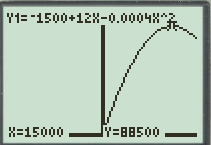# The maximum profit and number of units sold to generate it.### Precalculus: Mathematics for Calcu...

6th Edition
Stewart + 5 others
Publisher: Cengage Learning
ISBN: 9780840068071### Precalculus: Mathematics for Calcu...

6th Edition
Stewart + 5 others
Publisher: Cengage Learning
ISBN: 9780840068071

#### Solutions

Chapter 2, Problem 70RE
To determine

## To find: The maximum profit and number of units sold to generate it.

Expert Solution

The maximum profit is equal to 88500 and number of units sold to generate it is equal to 15000 .

### Explanation of Solution

Given information:

The profit P (in dollars) generated by selling x units of a certain commodity is given by P(x)=1500+12x0.0004x2 .

Calculation:

Graph the profit function P(x)=1500+12x0.0004x2 .

To graph a function y=1500+12x0.0004x2 , follow the steps using graphing calculator.

First press “ON” button on graphical calculator, press Y= key and enter right hand side of the equation y=1500+12x0.0004x2 after the symbol Y1 . Enter the keystrokes ()1500+12X0.0004X^2 .

The display will show the equation,

Y1=1500+12X0.0004X^2

Press the window key and adjust the window to [20000,20000] to [10,100000] .

Now, press the GRAPH key and TRACE key to produce the graph of given function in standard window as shown in Figure (1).Figure (1)

The maximum value of the profit function is 88500 at x=15000 .

Therefore, the maximum profit is equal to 88500 and number of units sold to generate it is equal to 15000 .

### Have a homework question?

Subscribe to bartleby learn! Ask subject matter experts 30 homework questions each month. Plus, you’ll have access to millions of step-by-step textbook answers!